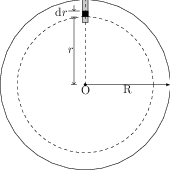# Law of Gravitation

## Problems from IIT JEE

Problem (IIT JEE 2014): A planet of radius $R=\frac{1}{10}\times (\text{radius of Earth})$ has the same mass density as Earth. Scientists dig well of depth $\frac{R}{5}$ on it and lower a wire of the same length and of linear mass density ${10}^{-3}\;\mathrm{kg/m}$ into it. If the wire is not touching anywhere, the force applied at the top of the wire by a person holding it in place is, (take the radius of Earth $={6\times{10}^{6}}\;\mathrm{m}$ and the acceleration due to gravity on Earth $={10}\;\mathrm{m/s^2}$.

1. 96 N
2. 108 N
3. 120 N
4. 150 N

Solution:Given $R_e=10R=6\times{10}^{6}\;\mathrm{m}$ and $g_e={10}\;\mathrm{m/s^2}$. Consider a wire element of length $\mathrm{d}r$ placed at a distance $r$ from the centre O (see figure). Let $\rho$ be common mass density of the planet and the earth and $m$ be mass of the planet inside the sphere of radius $r$ i.e., $m=\frac{4}{3}\pi r^3 \rho$. The gravitational force on the wire element by the planet is equal to the force by a point mass of magnitude $m$ placed at the centre O. Thus, force on the wire element is, \begin{align} \mathrm{d}F=\frac{Gm\lambda\mathrm{d}r}{r^2}=\frac{4}{3}G\pi\rho \lambda r\mathrm{d}r.\nonumber \end{align} Integrate $\mathrm{d}F$ from $r=\frac{4}{5}R$ to $r=R$ to get, \begin{align} F&=\frac{4}{3}G\pi\rho\lambda\int_{\frac{4}{5}R}^{R} r\mathrm{d}r=\frac{4}{3}G\pi \rho\lambda \left(\frac{9R^2}{50}\right)=\frac{\frac{4}{3}\pi\rho R_e^3 G}{R_e^2}\left(\frac{9\lambda R_e}{5000}\right)\nonumber\\ &=g_e \frac{9\lambda R_e}{5000}=\frac{(10)(9)({10}^{-3})(6\times{10}^{6})}{5000}={108}\;\mathrm{N}.\nonumber \end{align}